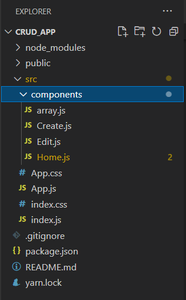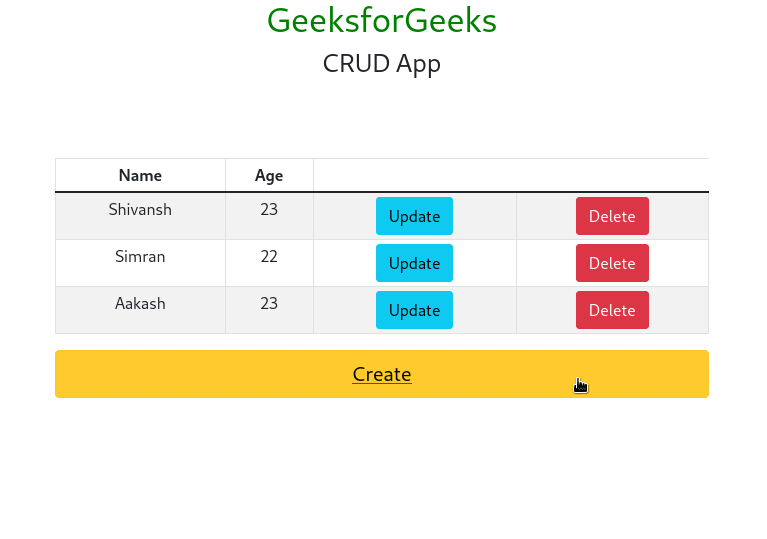# How to do CRUD operations in ReactJS ?

CRUD operation in React stands for Create, Read, Update, and Delete. CRUD is an important concept for organizing and managing data across the web application. We will perform CRUD operation in the React application with local storage in the form of JavaScript Objects instead of using JSON servers or Axios in React. CRUD operations are referred to as

• Create: Creating a post or adding the table row, adding data to the webpage, or creating content.
• Update: Updating or editing existing content on the webpage
• Delete: Deleting and removing the entry or content/post from the webpage

## Approach

To implement CRUD operations in React JS on locally stored data, use a data file array.js and create different components home, create, edit and link them with the data present in the js file. Access the data from the JavaScript Localstorage API . Add routing for these components using react-router-dom ann style using the React-Bootstrap UI components and Bootstrap style classes.

Steps to create the application

Step 1: Let’s start building the Front-end part with React. To create a new React App, enter the following code into the terminal and hit enter.

`npx create-react-app crud_app`

Step 2: Move into the React project folder.

`cd crud_app`

Step 3: Install other dependencies using this command

`npm i react-bootstrap bootstrap@5.1.3 react-router-dom`

Project Structure:Updated dependencies in package.json file will look like:

`"dependencies": {        "@testing-library/jest-dom": "^5.17.0",        "@testing-library/react": "^13.4.0",        "@testing-library/user-event": "^13.5.0",        "bootstrap": "^5.1.3",        "react": "^18.2.0",        "react-bootstrap": "^2.9.0",        "react-dom": "^18.2.0",        "react-router-dom": "^6.16.0",        "react-scripts": "5.0.1",        "web-vitals": "^2.1.4"}`

Example: This example show CRUD operations on a locally stored data file named array.js using React JS.

## Javascript

 `// Filename - App.js`   `import React from ``"react"``;` `import {` `    ``BrowserRouter as Router,` `    ``Route,` `    ``Routes,` `} from ``"react-router-dom"``;` `import ``"./App.css"``;` `import Create from ``"./components/Create"``;` `import Edit from ``"./components/Edit"``;` `import Home from ``"./components/Home"``;`   `function` `App() {` `    ``return` `(` `        ``
` `            ``

GeeksforGeeks

` `            ``

CRUD App

` `            ``` `                ``` `                    ``} />` `                    ``}` `                    ``/>` `                    ``}` `                    ``/>` `                ``` `            ``` `        ``
` `    ``);` `}`   `export ``default` `App;`

## Javascript

 `// Filename - components/array.js`   `// Javascript object named array ` `// with 3 key-values` `const array = [` `    ``{` `        ``id: ``"1"``,` `        ``Name: ``"Shivansh"``,` `        ``Age: ``"23"``,` `    ``},` `    ``{` `        ``id: ``"2"``,` `        ``Name: ``"Simran"``,` `        ``Age: ``"22"``,` `    ``},` `    ``{` `        ``id: ``"3"``,` `        ``Name: ``"Aakash"``,` `        ``Age: ``"23"``,` `    ``},` `];`   `export ``default` `array;`

## Javascript

 `// Filename - components/Create.js`   `import React, { useState } from ``"react"``;` `import { Button, Form } from ``"react-bootstrap"``;` `import ``"bootstrap/dist/css/bootstrap.min.css"``;` `import array from ``"./array"``;` `import { v4 as uuid } from ``"uuid"``;` `import { Link, useNavigate } from ``"react-router-dom"``;`   `function` `Create() {` `    ``// Making usestate for setting and` `    ``// fetching a value in jsx` `    ``const [name, setname] = useState(``""``);` `    ``const [age, setage] = useState(``""``);`   `    ``// Using useNavigation for redirecting to pages` `    ``let history = useNavigate();`   `    ``// Function for creating a post/entry` `    ``const handelSubmit = (e) => {` `        ``e.preventDefault(); ``// Prevent reload`   `        ``const ids = uuid(); ``// Creating unique id` `        ``let uni = ids.slice(0, 8); ``// Slicing unique id`   `        ``// Fetching a value from usestate and` `        ``// pushing to javascript object` `        ``let a = name,` `            ``b = age;` `        ``if` `(name == ``""` `|| age == ``""``) {` `            ``alert(``"invalid input"``);` `            ``return``;` `        ``}` `        ``array.push({ id: uni, Name: a, Age: b });`   `        ``// Redirecting to home page after creation done` `        ``history(``"/"``);` `    ``};`   `    ``return` `(` `        ``
` `            ``` `                ``{``/* Fetching a value from input textfirld ` `                    ``in a setname using usestate*/``}` `                ``` `                    ``` `                            ``setname(e.target.value)` `                        ``}` `                        ``type=``"text"` `                        ``placeholder=``"Enter Name"` `                        ``required` `                    ``/>` `                ```   `                ``{``/* Fetching a value from input textfirld in` `                    ``a setage using usestate*/``}` `                ``` `                    ``` `                            ``setage(e.target.value)` `                        ``}` `                        ``type=``"number"` `                        ``placeholder=``"Age"` `                        ``required` `                    ``/>` `                ```   `                ``{``/* handing a onclick event in button for` `                    ``firing a function */``}` `                `` handelSubmit(e)}` `                    ``variant=``"primary"` `                    ``type=``"submit"` `                ``>` `                    ``Submit` `                ```   `                ``{``/* Redirecting back to home page */``}` `                ``` `                    ``` `                ``` `            ``` `        ``
` `    ``);` `}`   `export ``default` `Create;`

## Javascript

 `// Filename - components/Home.js`   `import React from ``"react"``;` `import { Button, Table } from ``"react-bootstrap"``;` `import ``"bootstrap/dist/css/bootstrap.min.css"``;` `import array from ``"./array"``;` `import { Link, useNavigate } from ``"react-router-dom"``;`   `function` `Home() {` `    ``let history = useNavigate();`   `    ``// You may skip this part if you're` `    ``// using react-context api or redux` `    ``function` `setID(id, name, age) {` `        ``localStorage.setItem(``"id"``, id);` `        ``localStorage.setItem(``"Name"``, name);` `        ``localStorage.setItem(``"Age"``, age);` `    ``}`   `    ``// Deleted function - functionality` `    ``// for deleting the entry` `    ``function` `deleted(id) {` `        ``let index = array` `            ``.map(``function` `(e) {` `                ``return` `e.id;` `            ``})` `            ``.indexOf(id);`   `        ``// deleting the entry with index` `        ``array.splice(index, 1);`   `        ``// We need to re-render the page for getting` `        ``// the results so redirect to same page.` `        ``history(``"/"``);` `    ``}`   `    ``return` `(` `        ``
` `            ``` `                ``
NameAge
` `                    ``` `                        ``` `                        ``` `                    ``` `                ``` `                ``
{item.Name}{item.Age}` `                                    ``` `                                        ``` `                                                ``setID(` `                                                    ``item.id,` `                                                    ``item.Name,` `                                                    ``item.Age` `                                                ``)` `                                            ``}` `                                            ``variant=``"info"` `                                        ``>` `                                            ``Update` `                                        ``` `                                    ``` `                                ``` `                                    ``` `                                            ``deleted(item.id)` `                                        ``}` `                                        ``variant=``"danger"` `                                    ``>` `                                        ``Delete` `                                    ``` `                                ``
` `                    ``{``/* Mapping though every element ` `                        ``in the array and showing the ` `                        ``data in the form of table */``}` `                    ``{array.map((item) => {` `                        ``return` `(` `                            ``` `                                ``` `                                ```   `                                ``{``/* getting theid,name, and ` `                                    ``age for storing these` `                                    ``value in the jsx with ` `                                    ``onclick event */``}` `                                ```   `                                ``{``/* Using thr deleted function passing` `                                    ``the id of an entry */``}` `                                ``` `                            ``` `                        ``);` `                    ``})}` `                ``` `            ```   `            ``{``/* Button for redirecting to create page for` `                ``insertion of values */``}` `            ``` `                ``` `            ``` `        ``` `    ``);` `}`   `export ``default` `Home;`

## Javascript

 `// Filename - Edit.js` `import React, { useEffect, useState } from ``"react"``;` `import { Button, Form } from ``"react-bootstrap"``;` `import ``"bootstrap/dist/css/bootstrap.min.css"``;` `import array from ``"./array"``;` `import { Link } from ``"react-router-dom"``;` `import { useNavigate } from ``"react-router-dom"``;`   `function` `Edit() {` `    ``// Here usestate has been used in order` `    ``// to set and get values from the jsx` `    ``const [name, setname] = useState(``""``);` `    ``const [age, setage] = useState(``""``);` `    ``const [id, setid] = useState(``""``);`   `    ``// Used for navigation with logic in javascript` `    ``let history = useNavigate();`   `    ``// Getting an index of an entry with an id` `    ``let index = array` `        ``.map(``function` `(e) {` `            ``return` `e.id;` `        ``})` `        ``.indexOf(id);`   `    ``// Function for handling the edit and` `    ``// pushing changes of editing/updating` `    ``const handelSubmit = (e) => {` `        ``// Preventing from reload` `        ``e.preventDefault();` `        ``if` `(name == ``""` `|| age == ``""``) {` `            ``alert(``"invalid input"``);` `            ``return``;` `        ``}`   `        ``// Getting an index of an array` `        ``let a = array[index];`   `        ``// Putting the value from the input` `        ``// textfield and replacing it from` `        ``// existing for updation` `        ``a.Name = name;` `        ``a.Age = age;` `      `    `        ``// Redirecting to main page` `        ``history(``"/"``);` `    ``};`   `    ``// Useeffect take care that page will` `    ``// be rendered only once` `    ``useEffect(() => {` `        ``setname(localStorage.getItem(``"Name"``));` `        ``setage(localStorage.getItem(``"Age"``));` `        ``setid(localStorage.getItem(``"id"``));` `    ``}, []);`   `    ``return` `(` `        ``
` `            ``` `                ``{``/* setting a name from the ` `                    ``input textfiled */``}` `                ``` `                    ``` `                            ``setname(e.target.value)` `                        ``}` `                        ``type=``"text"` `                        ``placeholder=``"Enter Name"` `                    ``/>` `                ```   `                ``{``/* setting a age from the input textfiled */``}` `                ``` `                    ``` `                            ``setage(e.target.value)` `                        ``}` `                        ``type=``"number"` `                        ``placeholder=``"Age"` `                    ``/>` `                ```   `                ``{``/* Hadinling an onclick event ` `                    ``running an edit logic */``}` `                `` handelSubmit(e)}` `                    ``variant=``"primary"` `                    ``type=``"submit"` `                    ``size=``"lg"` `                ``>` `                    ``Update` `                ```   `                ``{``/* Redirecting to main page after editing */``}` `                ``` `                    ``` `                ``` `            ``` `        ``
` `    ``);` `}`   `export ``default` `Edit;`

## CSS

 `/* App.css */` `.App {` `    ``text-align``: ``center``;` `}` `.geeks {` `    ``color``: ``green``;` `}`

Steps to run the application:

Step 1: Use this npm command in project directory to run the applicaion.

`npm start `

Output: This output will be visible on http://localhost:3000/The CRUD operations in React JS are performed on Local Storage, for learning CRUD operation with ReactJS and NodeJS please refer to How to build a basic CRUD app with Node.js and ReactJS?

Whether you're preparing for your first job interview or aiming to upskill in this ever-evolving tech landscape, GeeksforGeeks Courses are your key to success. We provide top-quality content at affordable prices, all geared towards accelerating your growth in a time-bound manner. Join the millions we've already empowered, and we're here to do the same for you. Don't miss out - check it out now!

Previous
Next